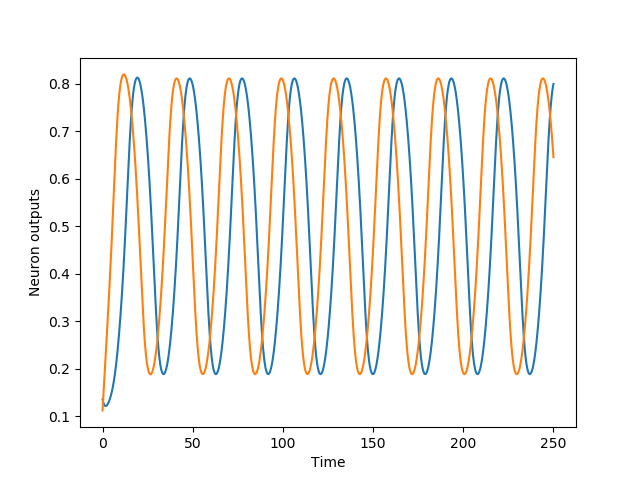Skip to content

# madvn/CTRNN

Python package that implements Continuous Time Recurrent Neural Networks (CTRNNs)
Latest commit 109f9fd Feb 26, 2019
Type Name Latest commit message Commit time
Failed to load latest commit information.CTRNNdemotestsLICENSEMANIFEST.inREADME.mdsetup.py

# CTRNN

Python package that implements Continuous Time Recurrent Neural Networks (CTRNNs)

See Beer, R.D. (1995). On the dynamics of small continuous-time recurrent neural networks. Adaptive Behavior 3:469-509. for a study of CTRNNs.

## Installation instructions

``````    \$ pip install CTRNN
``````

Requirements: numpy, scipy

## Using the package

#### Importing the CTRNN package:

``````    from CTRNN import CTRNN
``````

#### Creating a CTRNN object:

``````    cns = CTRNN(network_size,step_size=0.1)
``````

weights are initialized randomly; gains, time-constants and biases are set to 1

#### Setting gain for neuron i:

``````    cns.gains[i] = 1
``````

where i is in range [0,network_size)

#### Setting gain for all neurons:

``````    cns.gains = [1,2,3,..]
``````

with list of size=network_size

#### Setting biases and time-constants (taus) is similar

``````    cns.biases
cns.taus
``````

#### Setting weights to neuron i from neuron j:

``````    cns.weights[i,j] = 3
``````

where i,j in range [0,network_size)

#### Setting weights as a matrix:

``````    from scipy.sparse import csr_matrix
cns.weights = csr_matrix(weights_matrix)
``````

where weights_matrix is of size=network_sizeXnetwork_size

#### Euler stepping the network:

``````    cns.euler_step(external_inputs)
``````

where external_inputs is a list of size=network_size

#### Accessing/Setting output of neuron i:

``````    print(cns.outputs[i])
cns.outputs[i] = 0.5
``````

where i in range [0,network_size) and output in range [0,1]

#### Accessing/Setting output of all neurons:

``````    print(cns.outputs)
cns.outputs = [0.5,0.75,0.4]
``````

where list is of size=network_size

#### Same as above for states

``````    cns.states
``````

where state values can range in (-inf,inf)

#### Randomizing states/outputs

``````    cns.randomize_states(ub,lb)
``````

upper bound and lower bound in range (-inf,inf)

``````    cns.randomize_outputs(ub,lb)
``````

upper bound and lower bound in [0,1]

## Example

The following code creates a 2-neuron CTRNN sinusoidal oscillator, See demo folder::

``````    # imports
import numpy as np
import matplotlib.pyplot as plt
# importing the CTRNN class
from CTRNN import CTRNN

# params
run_duration = 250
net_size = 2
step_size = 0.01

# set up network
network = CTRNN(size=net_size,step_size=step_size)
network.taus = [1.,1.]
network.biases = [-2.75,-1.75]
network.weights[0,0] = 4.5
network.weights[0,1] = 1
network.weights[1,0] = -1
network.weights[1,1] = 4.5

# initialize network
network.randomize_outputs(0.1,0.2)

# simulate network
outputs = []
for _ in range(int(run_duration/step_size)):
network.euler_step(*net_size) # zero external_inputs
outputs.append([network.outputs[i] for i in range(net_size)])
outputs = np.asarray(outputs)

# plot oscillator output
plt.plot(np.arange(0,run_duration,step_size),outputs[:,0])
plt.plot(np.arange(0,run_duration,step_size),outputs[:,1])
plt.xlabel('Time')
plt.ylabel('Neuron outputs')
plt.show()
``````

Output:You can’t perform that action at this time.